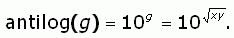SEARCH HOMEMath Central Quandaries & QueriesQuestion from Jack, a parent: Would like to know the basic geometric mean calculation.  Also how would I take the antilog from this this number?Hi Jack.

The geometric mean (sometimes called the "mean proportional") is the number between two other numbers which makes the ratio of the first to the geometric mean the same as the ratio of the geometric mean to the second. So if you have two numbers x and y and want the geometric mean, you have:

x/g = g/y

which is the same as saying

g = (xy)½ = SQRT(xy).

For example, if you want the geometric mean of 8 and 72, you would have SQRT(8×72) = 24.

The antilog of a number is the opposite of taking a logarithm of a number. Generally, we use it with base 10 logs, so both of the following are true:

log10(100) = 2 and

antilog10(2) = 100.

Basically then, taking the antilog of a number is raising 10 to its power. So the antilog of the geometric mean isCheers,
Stephen La Rocque.Math Central is supported by the University of Regina and The Pacific Institute for the Mathematical Sciences.